# Ages 2

A man's age is 4 times his son's age. After 5 years he will be just twice his son's age, find their ages.

Correct result:

f =  10
s =  2.5

#### Solution:

f = 4s
f+5 = 2(s+5)

f = 4•s
f+5 = 2•(s+5)

f-4s = 0
f-2s = 5

f = 10
s = 5/2 = 2.5

Our linear equations calculator calculates it.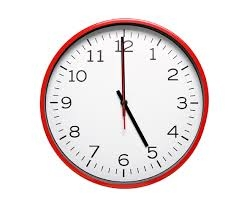We would be pleased if you find an error in the word problem, spelling mistakes, or inaccuracies and send it to us. Thank you!Tips to related online calculators
Do you have a system of equations and looking for calculator system of linear equations?
Do you want to convert time units like minutes to seconds?

## Next similar math problems:

• Mother and sonMother is four times older than her son. In 16 years, the son will be two times younger than his mother. How many years are mother and son?
• Age problemsA) Alex is 3 times as old as he was 2 years ago. How old is he now? b) Casey was twice as old as his sister 3 years ago. Now he is 5 years older than his sister. How old is Casey? c) Jessica is 4 years younger than Jennifer now. In 10 years, Jessica will
• The agesThe ages of the four sons make an arithmetic sequence, the sum of which is the age of the father today. In three years, the father's age will be the sum of the ages of the three eldest sons, and in the next two years and three months, the father's age wil
• Father 7Father is six times older than his son. After four years, the father will only be four times older. What are their present ages?
• Father and son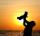Father is three times older than his son. Twelve years ago, the father was nine times more senior than the son. How old are father and son?
• Family and age ratiosAge of father and son are in the ratio 10:3; age of father and daughter are in the ratio 5:2. How old is father and son, if daughter is 20 years old? In what ratio is age sister and brother?
• CarlaCarla is 5 years old and Jim is 13 years younger than Peter. One year ago, Peter’s age was twice the sum of Carla’s and Jim’s age. Find the present age of each one of them.
• Father and son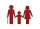When I was eight years old, my father was 30 years old. Today, my father is 3 times more than me. How old am I?
• Daughters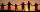The man conducting the census asks a woman to age of three daughters. Woman says when multiply the age getnumber 72; if their ages add up, get a number of our house, as you see. The man says: That is not enough to calculate their ages. She says: my oldest
• Father and sonWhen I was 11, my father was 35 years old. Today, my father has three times more years than me. How old is she?
• 14 yearsKája is 14 years old. Mom 44. How many years will mom be 4 times older?
• Father and sonFather and son weigh together 108kg. The father weighs 2.6 times more than his son. How much does weight my father and son count?
• Lee isLee is 8 years more than twice Park's age, 4 years ago, Lee was three times as old. How old was Lee 4 years ago?
• Two ages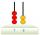Gabrielle is 9 years younger than Mikhail. The sum of their ages is 87. What is Mikhail's age?
• A man 2A man divides \$10,000 into two investments, one at 10% and the other at 30%. Find how much is invested at each rate so that the two investments produce the same income annually.
• Mother and daughter 2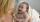The mother is 40 years older than her daughter. How old is the mother if her age is eight thirds age of daughter?
• Father and son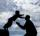Father is 44 years old, his son 16 years. Determine how many years ago was the father five times older than the son.